#Rule 25: Complex FractionsTo multiply two complex fractions, convert the fractions to simple fractions and follow the steps you use to multiply two simple fractions.Example:
Calculate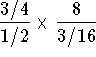.
Solution 1:
Convert the numerator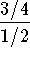to a simple fraction.can be written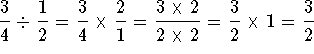.

Convert the denominator to a simple fraction.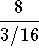can be written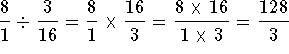. The problemcan now be written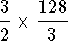. Multiply the numerators and multiply the denominators.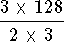. The problem is reduced by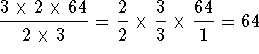.

Solution 2:
Convert the 8 to a fraction, multiply the numerators, and multiply the denominators.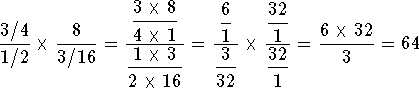.

### If you want to review another example, click on the word Example. Work the following problems and click on Answer if you want to check the answer and review the solution.

Problem 1: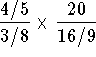.

Problem 2: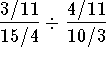.

Problem 3: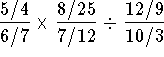.[Simple Fractions] [Complex Fractions] [Compound Fractions]
[Decimals] [Percentage] [Rules]

[Algebra] [Trigonometry] [Complex Variables]S.O.S MATHematics home page

Do you need more help? Please post your question on our S.O.S. Mathematics CyberBoard.Author: Nancy Marcus